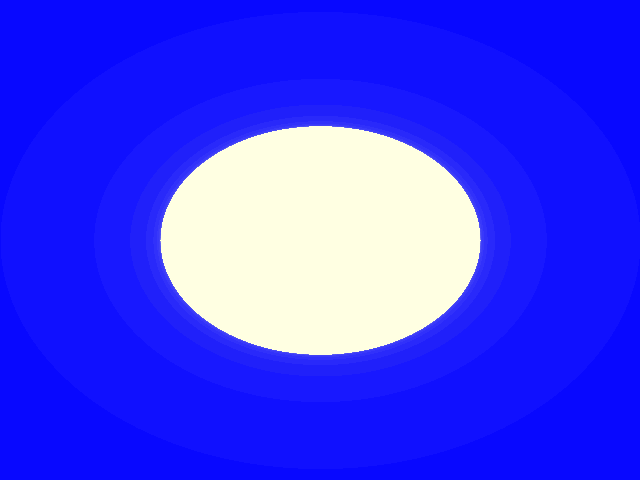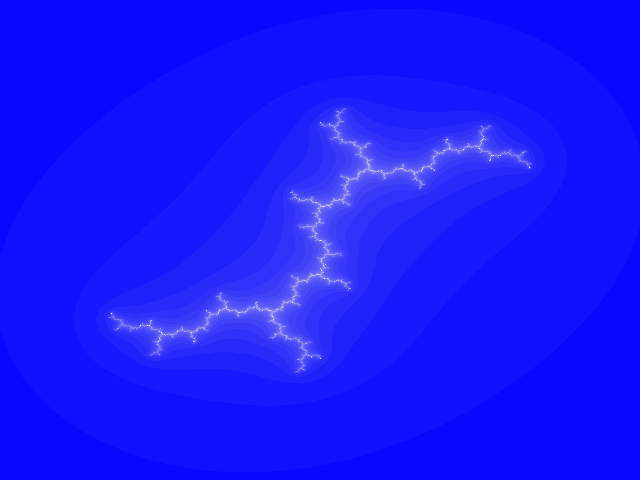## Saturday, February 1, 2014

### Animated Javascript Julia fractals

Let us start from something basic. Let's take x with the initial value of 1. The next value will be computed by multiplying the previous value by 2. This gives us a new x and we apply the same rule to it.

More formally,
```        x0 = 1
xn+1 = 2 * xn
```
gives us the sequence: 1, 2, 4, 8, 16, 32 ...

What if the initial value was not 1 but, for example, 1.0001? The second value would be 2.0002, then 4.0004, 8.0008, 16.0016 ... The sequence is predictable, although we have started from different initial value, we can easily predict the n-th value.

Let's examine another sequence:
```        xn+1 = 4 * xn * (1-xn)
```
If an initial value is from the [0,1] range, all sequence values also belong to [0,1] (why?). Similarily to what we have done before, let us examine two sequences starting with two different but close initial values, for example 0.2 i 0.2000001. We will see how sequences are related.

Few initial iterations don't reveal anything suspicious:
``` xa=0.2000000, xb=0.2000001, difference=0.0000001
xa=0.6400000, xb=0.6400002, difference=0.0000002
xa=0.9216000, xb=0.9215997, difference=0.0000003
xa=0.2890138, xb=0.2890147, difference=0.0000009
xa=0.8219392, xb=0.8219408, difference=0.0000015
xa=0.5854205, xb=0.5854166, difference=0.0000039
xa=0.9708133, xb=0.9708160, difference=0.0000027
xa=0.1133392, xb=0.1133291, difference=0.0000101
```
... but somewhere around 20-th iteration we have
``` ...
xa=0.8708927, xb=0.0382300, błąd=0.8326627
xa=0.4497544, xb=0.1470737, błąd=0.3026806
xa=0.9899015, xb=0.5017722, błąd=0.4881293
xa=0.0399860, xb=0.9999874, błąd=0.9600014
xa=0.1535486, xb=0.0000503, błąd=0.1534983
...
```
Both sequences seem unrelated at all. A disaster!

Such behavior of sequences is called chaotic. The discovery of chaotic sequences was quite a shock for scientists who believed that this is only a matter of time to create formulas for all laws of nature. It turned out however that many simple formulas behave in such chaotic way.

Imagine that we would like to predict weather. We'd like to have a formula that given some measured values (temperature, atmospheric pressure, humidity) computes the temperature for tomorrow and then next week or next year.

For years scientists believed this is possible. However, our simple experiment proves that if such hypothetical formula would be chaotic (and would it be?) then the formula could possibly give quite a good prediction for the very next day and the day after but could completely fail to predict weather for 20th day and later.

But the story continues. Such simple sequences could have a beautiful graphical interpretation - fractals! Such fractals are parts of the world that surrounds us, just like atoms. And unlike atoms - which are not eternal - fractals are perfect and eternal.

Fractal Julia Sets "live" on the two-dimensional plane. They are graphical interpretations of a following sequence:
```        x0 = coordinates of a point from the plane
xn+1 = xn * xn + c       (1)
```
(where c is a fixed complex number)

We assume that the fractal image is the [-2,2] x [-2,2] subset of the comple plane. Each point from the image corresponds to one and only one point from the subset (with a simple linear correspondence).

Well then, we have a point, the x. How do we compute x * x? What does it mean to multiply point by a point?

Well, each point from a plane can be interpreted as a complex number. Complex numbers can be added, subtracted, multiplied and divided. For example, a square of point (1,1) is (0,2), and (1,2)*(2,-4) gives (10,0).

The algorithm of generating a Julia set consist in computing the sequence (1) for each point of our complex subplane. The sequence either reaches a bounded orbit or values "run away" to infinity.

We color points that give a bounded orbit as black and points that "run away" to infinity as white.

And that's all. We can create our very first Julia set for the c of the definition (1) equal to 0 (complex 0 + 0i).Wow, a circle! Is this even a fractal? Well, let's have another Julia for c equal to (0,1) (complex 0 + 1i).What happened to the circle? Well, this is a perfect example of chaos. Those points of the complex subplane that give sequences with bound orbits create the dendrite.

Another interesting thing about Julias is that these sets are symmetric, consist of two identical parts reflected by the origin of the plane. This is not a coincidence - a sequence x -> x * x * x + c would give three symmetric parts, x -> x^4 + c - four and so on.

The code:
`\$.noConflict();`
` `
`var cx = 0, cy = 0, kat1 = 0, kat2 = 1;`
`var WJulia = 768;`
`var HJulia = 768;`
`var contextJulia;`
`var pixJulia;`
`var imgdJulia;`
`var idJulia;`
`var frame = 0;`
` `
`function SetupJulia()`
`{`
`    clearInterval( idJulia );`
` `
`    var elemJulia = document.getElementById('JuliaCanvas');`
`    if (elemJulia && elemJulia.getContext)`
`    {`
`        contextJulia = elemJulia.getContext('2d');`
`        if (contextJulia)`
`        {`
`            if (contextJulia.createImageData)`
`                imgdJulia = contextJulia.createImageData(WJulia, HJulia);`
`            else`
`                imgdJulia = contextJulia.getImageData(0, 0, WJulia, HJulia);`
` `
`            pixJulia = imgdJulia.data;`
`        }`
`    }`
` `
`    idJulia = setInterval(LoopJulia, 3);`
`}`
` `
`function LoopJulia()`
`{`
` `
`    kat1 += 0.003;`
`    kat2 += 0.007;`
`    cx = 981 * Math.sin(kat1);`
`    cy = 983 * Math.cos(kat2);`
`    frame++;`
` `
`    /* tworzenie bitowego obrazu */`
`    RysujJulie();`
` `
`    /* kopiowanie bitowego obrazu do context/canvas */`
`    contextJulia.putImageData(imgdJulia, 0, 0);`
`    contextJulia.font = "bold 12px sans-serif";`
`    contextJulia.fillStyle = 0;`
`    contextJulia.fillText( frame, 20, 20 );`
`}`
` `
` `
`function RysujJulie()`
`{`
`    var px = 0;`
`    for (var i = -2304; i < 2304; i = i + 6)`
`    {`
`        var py = 0;`
`        for (var j = -2304; j < 2304; j = j + 6)`
`        {`
`            var c = 0;`
`            var x = i;`
`            var y = j;`
`            var x2 = x * x;`
`            var y2 = y * y;`
` `
`            while (((x2 + y2) < 4000000) && (c < 31))`
`            {`
`                c++;`
` `
`                y  = ((x * y) >> 9) + cy;`
`                x  = ((x2 - y2) >> 10) + cx;`
`                x2 = x * x;`
`                y2 = y * y;`
` `
`            }`
` `
`            SetPixelColor( pixJulia, (py * WJulia + px) << 2, `
`              255, 255-(8*c), 255-(6 * c), 255 - c );`
` `
`            py++;`
`        }`
` `
`        px++;`
`    }`
`}`
` `
`function SetPixelColor(pix,offs, a, r, g, b)`
`{            `
`    pix[offs++] = r;`
`    pix[offs++] = g;`
`    pix[offs++] = b;`
`    pix[offs] = a;`
`}`
` `
`jQuery(function() {`
`  SetupJulia();`
`});`

Enough theory, let's see it moving.